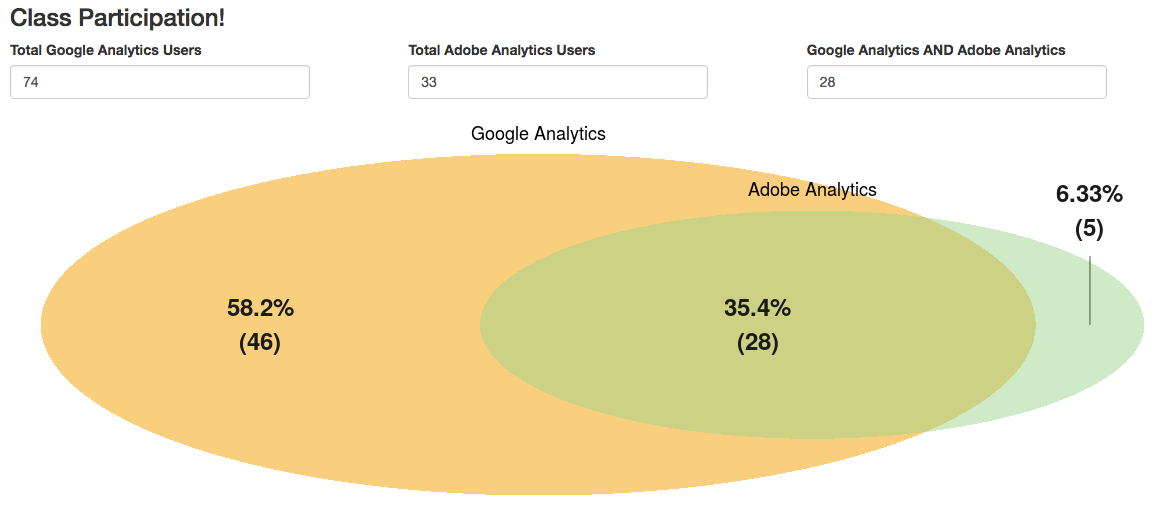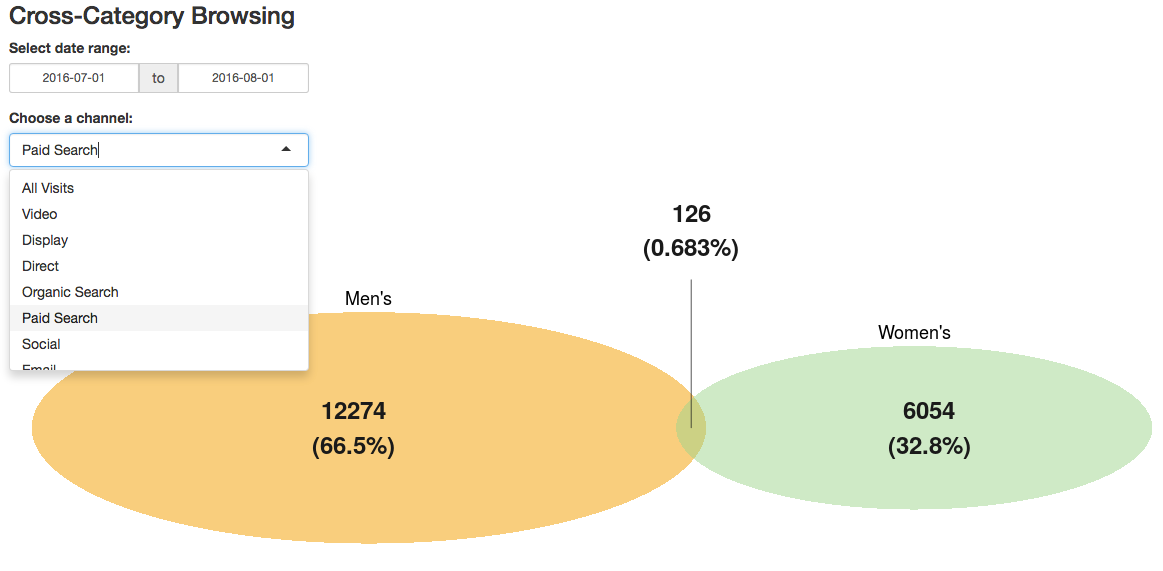This is an example of a visualization built using shiny (web-enablement) that was then repurposed for an entirely different use.

The first example, shown below, is available at https://gilligan.shinyapps.io/eMetrics_Venn/, if you want to try it out.shiny apps historically required two files, although the package has been updated to enable using a single file.

## The Code

### server.R

The code that runs behind the scenes (note that most of the code is really just setting up the formatting options for the Venn diagram itself):

library(shiny)
library(VennDiagram)

shinyServer(function(input, output) {

output$vennDiagram <- renderPlot({ # Only plot the Venn diagram if the input values are legit (the input values # are all available and the intersection area isn't greater than either individual set). if(as.numeric(req(input$adobeGoogle)) <= as.numeric(req(input$adobe)) && as.numeric(req(input$adobeGoogle)) <= as.numeric(req(input$google))){ draw.pairwise.venn(area1 = as.numeric(input$google),
area2 = as.numeric(input$adobe), cross.area = as.numeric(input$adobeGoogle),
fill = c("#F29B05","#A1D490"),
ext.text = TRUE,
ext.percent = c(0.1,0.1,0.1),
ext.length = 0.6,
label.col = rep("gray10",3),
lwd = 0,
cex = 2,
fontface = rep("bold",3),
fontfamily = rep("sans",3),
cat.cex = 1.5,
cat.fontface = rep("plain",2),
cat.fontfamily = rep("sans",2),
cat.pos = c(0, 0),
print.mode = c("percent","raw")
)
}
})
})

### ui.R

The code for the user interface:

library(shiny)

shinyUI(fluidPage(

# Application title
titlePanel(h3("Class Participation!")),

# Sidebar with a slider input for number of bins
fluidRow(
),

# Show a plot of the generated distribution
fluidRow(
column(12,plotOutput("vennDiagram"))
)
)
)

## Repurposing to Use Segments

The example above, obviously, was just a demo for a conference presentation. But, the demo was actually inspired by a real-world use case, which is the visualization of two different segments of traffic to a site.

This example is available at https://gilligan.shinyapps.io/segmentsVenn/.

It shows the volume of sessions from each segment and the degree to which traffic fell in both segments (the overlapping area), and provides the user with two settings that trigger a re-query of the data and an update of the diagram:

• Changing the date range
• Choosing a channel